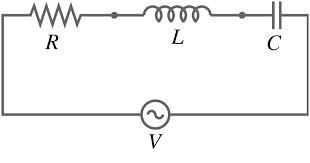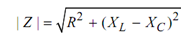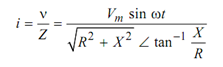## Series RLC Circuit Assignment Help

Assignment Help: >> Resistive - Inductive and Capacitive Circuits - Series RLC Circuit

Series RLC Circuit:

The applied voltage is v = Vm  sin ωt

Inductive reactance  X L  = ωL

Capacitive reactance X C  =1 / ωCFigure: Series RLC Circuit

Here, we describe the impedance of the circuit which is given by

Z = R + j ( X L  - X C )   in ohm

The magnitude of the impedance isand angle

θ= tan -1 (X L  - X C) / R

This is the angle between the applied voltage v and the current i. θ is also termed as the power factor angle of the circuit.

The current i is specified bywhere X = XL - XC

or        i = (Vm /| Z | ) sin  (ωt - tan -1 ( X /R)

If the circuit in inductive (XL > XC) then current i lags the v by angle tan -1 (X/ R)  . But if the circuit is capacitive then i leads the v by angle tan -1 (X/ R)  .

The voltage drop across each passive elements

VR = i R,           VL = XL i,           VC = XC i

Also       v¯ = v¯ R  + v¯ L  + v¯ C    (Kirchhoff's Voltage Law)

Generally, if we apply Kirchhoff's voltage law in series RLC circuit then KVL equation is given by

v = R i + L di/ dt +  (1 / C )∫ i dt